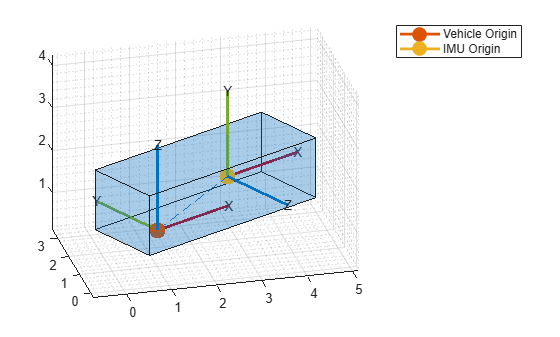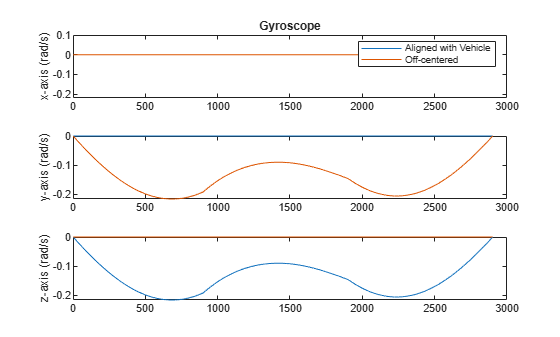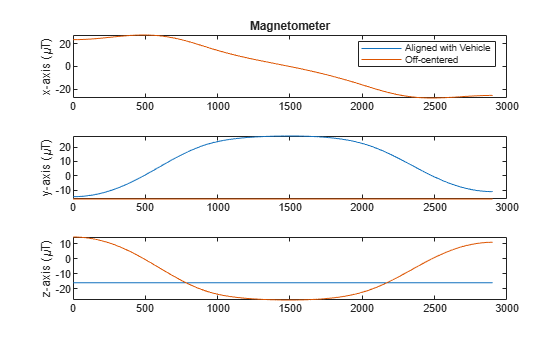This example shows how to generate inertial measurement unit (IMU) readings from a sensor that is mounted on a ground vehicle. Depending on the location of the sensor, the IMU accelerations are different.

### Create Trajectory

Specify the waypoint trajectory of a vehicle and compute the vehicle poses using `lookupPose`.

```% Sampling rate. Fs = 100; % Waypoints and times of arrival. waypoints = [1 1 1; 3 1 1; 3 0 0; 0 0 0]; t = [1; 10; 20; 30]; % Create trajectory and compute pose. traj = waypointTrajectory(waypoints, t, "SampleRate", Fs); [posVeh, orientVeh, velVeh, accVeh, angvelVeh] = lookupPose(traj, ... t(1):1/Fs:t(end));```

### Create Sensor and Define Offset

Create two 9-axis `imuSensor` objects composed of accelerometer, gyroscope, and magnetometer sensors. One `imuSensor` object generates readings of an IMU mounted at the vehicle's origin and the other one generates readings of an IMU mounted at the driver's seat. Next, specify the offset between the vehicle origin and the IMU mounted at the driver's seat. Call `helperPlotIMU` to visualize the locations of the sensors.

```% IMU at vehicle origin. imu = imuSensor("accel-gyro-mag", "SampleRate", Fs); % IMU at driver's seat. mountedIMU = imuSensor("accel-gyro-mag", "SampleRate", Fs); % Position and orientation offset of the vehicle and the mounted IMU. posVeh2IMU = [2.4 0.5 0.4]; orientVeh2IMU = quaternion([0 0 90], "eulerd", "ZYX", "frame"); % Visualization. helperPlotIMU(posVeh(1,:), orientVeh(1,:), posVeh2IMU, orientVeh2IMU);```### Calculate IMU Trajectory Using Vehicle Trajectory

Compute the ground truth trajectory of the IMU mounted at the driver's seat using the `transformMotion` function. This function uses the position and orientation offsets and the vehicle trajectory to compute the IMU trajectory.

```[posIMU, orientIMU, velIMU, accIMU, angvelIMU] = transformMotion( ... posVeh2IMU, orientVeh2IMU, ... posVeh, orientVeh, velVeh, accVeh, angvelVeh);```

Generate the IMU readings for both the IMU mounted at the vehicle origin and the IMU mounted at the driver's seat.

```% IMU at vehicle origin. [accel, gyro, mag] = imu(accVeh, angvelVeh, orientVeh); % IMU at driver's seat. [accelMounted, gyroMounted, magMounted] = mountedIMU( ... accIMU, angvelIMU, orientIMU);```

Compare the accelerometer readings of the two IMUs. Notice that the x-axis acceleration is different because of the off-center location.

```figure('Name', 'Accelerometer Comparison') subplot(3, 1, 1) plot([accel(:,1), accelMounted(:,1)]) legend('Aligned with Vehicle', 'Off-centered') title('Accelerometer') ylabel('x-axis (m/s^2)') subplot(3, 1, 2) plot([accel(:,2), accelMounted(:,2)]) ylabel('y-axis (m/s^2)') subplot(3, 1, 3) plot([accel(:,3), accelMounted(:,3)]) ylabel('z-axis (m/s^2)')```Compare the gyroscope readings of the two IMUs.

```figure('Name', 'Gyroscope Comparison') subplot(3, 1, 1) plot([gyro(:,1), gyroMounted(:,1)]) ylim([-0.22 0.1]) legend('Aligned with Vehicle', 'Off-centered') title('Gyroscope') ylabel('x-axis (rad/s)') subplot(3, 1, 2) plot([gyro(:,2), gyroMounted(:,2)]) ylabel('y-axis (rad/s)') subplot(3, 1, 3) plot([gyro(:,3), gyroMounted(:,3)]) ylabel('z-axis (rad/s)')```Compare the magnetometer readings of the two IMUs.

```figure('Name', 'Magnetometer Comparison') subplot(3, 1, 1) plot([mag(:,1), magMounted(:,1)]) legend('Aligned with Vehicle', 'Off-centered') title('Magnetometer') ylabel('x-axis (\muT)') subplot(3, 1, 2) plot([mag(:,2), magMounted(:,2)]) ylabel('y-axis (\muT)') subplot(3, 1, 3) plot([mag(:,3), magMounted(:,3)]) ylabel('z-axis (\muT)')```